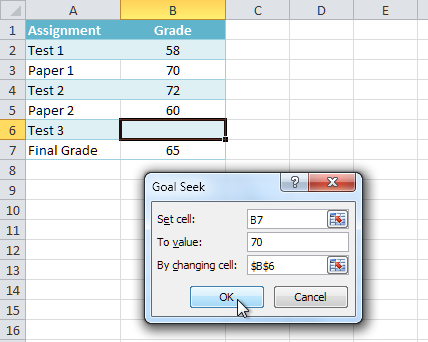# Select an option which properly completes the following: In Excel, the "What-If" analysis tool...

## Question:

Select an option which properly completes the following:

In Excel, the "What-If" analysis tool _____ cell values to achieve a desired outcome based on a target value.

(a) hides

(b) conditionally formats

(c) deletes

## "What-if" analysis :

"What-if" analysis allows you to change the values in cells so that you can see how those changes affect the formulas outcomes. What-if analysis is a useful way of being able to test out various scenarios in Excel. It allows you to try out different values (scenarios) for formulas. There are three kinds of what if analysis: Goal seek, scenario manager and data tables.You can look at these things in two different ways:

The first way is to change the input variables and see what impact that has on the output. Scenario manager and data tables work in this way and can answer questions like, what would happen to our profits if the number of units we sell doubled.

The second way is to say what outcome you would like to have and ask Excel to calculate what change in the inputs would be required to achieve this.The goal seek feature works this way and can answer questions such as how many units of a product need to be sold in order to reach a desired profit level.

"What-if" analysis allows you to change the values in cells so that you can see how those changes adjusts (affect) the formulas outcomes.

There are three kinds of what if analysis: Goal seek, scenario manager and data tables. The goal seek feature, we want desire outcome and ask Excel to calculate what change in the inputs would be required to achieve this. and can answer questions such as how many units of a product need to be sold in order to reach a desired profit level. Scenario manager and data tables, we change the input variables and see what impact that has on the output and can answer questions like, what would happen to our profits if the number of units we sell doubled.

Example: Suppose you're enrolled in a class. You currently have a grade of 65, and you need at least a 70 to pass the class. Luckily, you have one final assignment that might be able to raise your average. You can use Goal Seek to find out what grade you need on the final assignment to pass the class.